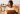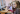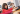# 6 Unexpected Benefits of Using Maths Worksheets

Almost every parent finds it difficult to teach mathematics at one stage. As we all know, mathematics is a complex subject; parents often look for solutions to overcome such problems. Maths worksheets are a great tool for implementing constant practice and vigor in children.Many platforms provide free math worksheets for children of all grades. The role of such educational resources is very prevalent in preschool education. Parents with children in the elementary grades are very familiar with these math worksheets as they often take them to school as homework. To teach the small children complex mathematical concepts like multiplication and division the math worksheet become very helpful. We can say it is a very handy tool to make your child more educated. These maths worksheets are a great way to keep kids constantly practicing learning challenging topics. By practicing math through worksheets, children can learn to interpret the various patterns and logic of mathematics.

Learning the fundamental aspects of mathematics ensures that children can work with different computational aspects, which ensures success in mathematics.

## Advantages of using maths worksheets

Here are six surprising benefits of using math sheets for kids:### Critical Thinking

Practicing math assignments allows children to apply and improve their problem-solving skills. This improves their logical thinking and ability to find creative solutions to various problems. By constantly working with math worksheets, children can easily work on their problem-solving skills to develop self-confidence. Maths worksheets are essential for primary education because they give children the opportunity to imagine everyday life problems in mathematical terms.

## Interactive training

Online math worksheets encourage students to learn better through simulation and interactive lessons. Instead of the usual paper worksheets, there is a more cautious approach to learning mathematics, such as mathematical games and applications.
Game interfaces are more convenient for children to effectively maintain the interest of the child. Developing a solid mathematical foundation can encourage children to structure strong conceptual knowledge on it.

## Facilitates time management

Some students often do poorly in math due to inefficient time management. To complete math assignments or exams they do not find many times. This type of inability leads to low grades or grades in math.

### Math worksheets make students solve problems faster than ever.

They become more confident in mathematics while maintaining the pace needed to solve mathematical problems. Over time, they also master some basic math skills and formulas to easily acquire mathematical skills. Such training allows children to solve various problems, better distributing time.

## Understanding concepts

Math worksheets encourage students to learn a variety of mathematical concepts, gradually working through each basic concept in detail. It provides a comprehensive study of each concept, allowing children to apply them to a variety of situations. Problem-solving, starting with the basics and gradually moving towards higher levels of complexity, allows children to learn basic concepts more effectively.

## Practice and revision

To practice basic math tasks the math worksheet becomes a great learning tool. It is also useful to understand basic mathematical concepts and patterns. Students can briefly use these math worksheets to improve their math knowledge through repeated practice. Practicing math assignments to correct math during exams helps students fully internalize concepts. It’s also a great way to give a clear outline of each topic to strengthen their exact math skills.

Math worksheets are a reliable way to practice for students at their own pace without stress and fear of failure. This is the perfect way to help students focus and improve their understanding of a particular topic.

## Get Focus

Math worksheets focus on fundamental concepts and complex topics that require more attention. By solving these worksheets, students easily learn to focus on the main topics of each chapter. Math worksheets improve children’s ability to focus on math while they learn the basic basics and step-by-step problem-solving.

Check out our Symphony Math program today.